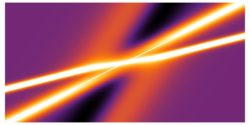### What is a soliton and an integrable system?

A soliton is a particular type of solution of a partial differential equation (PDEs) that has a particle-like behaviour. These particle-like solutions, called solitons may collide, but after collision they move on practically undisturbed. The existence of such solutions of an evolution equation is a very rare phenoThree-soliton solutions of the Korteweg-de Vries equation

menon, and only equations of a special type have soliton solutions. Although in general nonlinear PDEs are much harder to solve than linear ones, there are exact methods for finding soliton solutions such as the inverse scattering transform and Bäcklund transformations. Soliton equations have a number of important features that can be used to characterise them, including infinite families of symmetries ("commuting flows") and conservation laws, and Lax or zero curvature representations.

As well as soliton equations (such as the famous KdV equation), there are other classes of integrable systems. These include differential-difference equations describing nonlinear lattices (such as the Toda chain equation), Hamiltonian many-particle systems, spin models in statistical mechanics and Lagrangian models in quantum field theory. These are all interlinked through algebraic and geometric properties, some of which have led to entirely new concepts in pure and applied mathematics as well as in mathematical physics. Thus, although integrable systems appear to be very specialised they emerge in many areas of research, from water waves and plasma physics to the topology of knots and through the representation theory of infinite-dimensional Lie algebras. The Integrable Systems group has interest in a wide range of topics in this field.

A more detailed review can be found in A historical introduction to solitons and Bäcklund tranformations by A.P. Fordy, published in A.P. Fordy and J.C. Wood (Editors), Harmonic Maps and Integrable Systems. Vieweg, Wiesbaden, 1994.

A description of the group's current research interests can be found on the page Research Topics or on the individual pages of the members.

### Research Environment

The group has its own seminar series and frequently hosts Workshops and Conferences in Leeds. The post-graduate students of the group hold an informal seminar, in which they discuss various ideas and mathematical techniques which have arisen in their work.